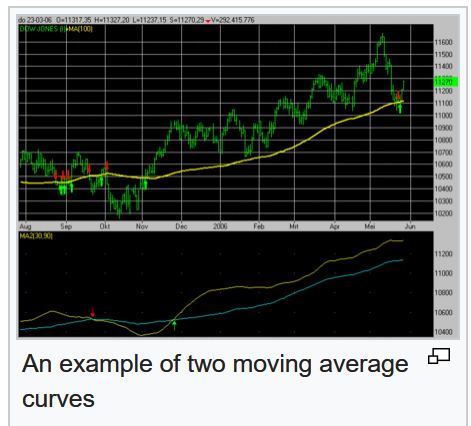# How many parameters in total we can learn in BatchNorm1d?

BatchNorm1d is not that obvious when I checked the source code.

Let’s check this:

``````if self.affine:
self.weight = Parameter(torch.Tensor(num_features))
self.bias = Parameter(torch.Tensor(num_features))
else:
self.register_parameter('weight', None)
self.register_parameter('bias', None)
``````

First `Parameter` is the instruction that `weight` will be the learnable parameter. This means we will learn the weight.

Then `register_parameter` adds a parameter to the module. But can you tell me what is the difference?

I just assume this has to do something with making parameters that are shared for every mini batch and that are specific for every mini batch.

There is also similar question without a clear answer.

The general question is: How many parameters in total we can learn in BatchNorm1d.
I think 4, but I am not sure.

I know if we set `affine=True` we will learn `weight` and `bias` parameters.

Apart from these two, I somehow think we can also learn `mean` and `std`. However, I am not sure.

Maybe the current implementation of `_BatchNorm` (which is the base class for `BatchNorm1d`) is not learning mean and std(var).

Here is the last bit of code I will share with you:

``````self.register_buffer('running_mean', torch.zeros(num_features))
self.register_buffer('running_var', torch.ones(num_features))
self.register_buffer('num_batches_tracked', torch.tensor(0, dtype=torch.long))
else:
self.register_parameter('running_mean', None)
self.register_parameter('running_var', None)
self.register_parameter('num_batches_tracked', None)
``````

From this code I can understand that we can track `running_mean` and `running_var` (which should be variance I guess) and number of mini batches (`num_batches_tracked`) we processed so far. The first two are tensors, and the second is a number of mini batches tracked. So at least these two are not the constants. Right?

Hi,
Check this post,

With respect to batch normalization the best you can do is to read the paper:

1 Like

I am providing this for my better understanding. So far I understood number of batches tracked will increase with every batch.

`````` if self.training and self.track_running_stats:
# TODO: if statement only here to tell the jit to skip emitting this when it is None
if self.num_batches_tracked is not None:
self.num_batches_tracked += 1
if self.momentum is None:  # use cumulative moving average
exponential_average_factor = 1.0 / float(self.num_batches_tracked)
else:  # use exponential moving average
exponential_average_factor = self.momentum
``````

`weight` and `bias` are learnable parameter of choice, and they can scale the output activation range effectively else it will be std (-1,1) as we saw with the mean of 0.

What are the running mean and running var?
They are used for the moving average calculation
Two types of moving averages we support as you can see:

• exponential moving average
• cumulative moving average

At the very end they will smoothen the curve which is what the batch norm do.So the running mean and running var are calculated as average, not learned like Parameter or some parameter in the nn.Linear module using the loss function.